# HRC RMF

### HRC-I | HRC-S | HRC/Cal

Note: This page describes the RMFs for use with PHA-based PI. As of CALDB 4.2/CIAO 4.2 (December 2009), the default is to calculate PI from scaled SUMAMPS (SAMP) rather than PHA. New RMFs have been created for use SAMP-based PI, see this page.

We have developed RMFs for the HRC-I and HRC-S. This page discusses the creation of and justification for the RMF and provides grids in hardness-ratio and quantile-width spaces for some common spectral models, as calculated with the new RMF.

## HRC-I

#### Introduction | Files | Grids

Vinay Kashyap & Jennifer Posson-Brown
November 2005

## Introduction

The intrinsic energy resolution of the HRC is poor compared with the ACIS, but it does have some ability to discriminate between hard and soft spectra. Lab tests of HRC-I pulse-height distributions obtained during sub-assembly calibration show a monotonic trend in the median PHA for line energies below 2 keV (see Figure 7.7 of the Chandra Proposers' Observatory Guide).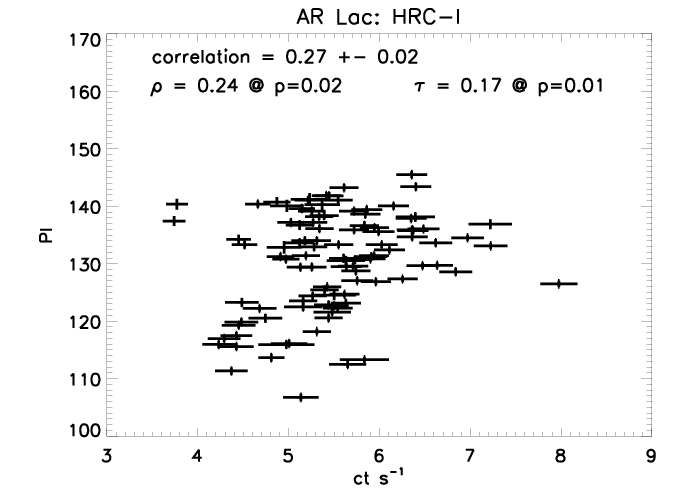This spectral discrimination is also seen in flight data. We have analyzed data from the flaring variable coronal source AR Lac, which has been regularly observed since 1999 as a calibration source to monitor the HRC-I gain and QE, and in the adjacent plot we show the count-rate and the corresponding median PI computed for each observation (each approximately 2 ks in duration) with off-axis values < 8 arcmin. When a coronal source flares, the plasma temperature is usually higher, and consequently the spectrum is harder; a (weak) correlation is therefore to be expected between count-rate and spectral hardness, and is indeed detected in the data. A correlation of 0.27 is observed, with an error of 0.02 determined after a Monte Carlo simulation of the individual errors. Pearson's coefficient rho is 0.24, with a p-value of 0.02, and Kendall's Rank correlation coefficient tau is 0.17, with a p-value of 0.01.

Using observations of HR1099 carried out using the HRC-I/LETG at various locations on the detector (ObsIDs 1388, 1389, 1392, 1393), we have constructed a response matrix for the HRC-I (see Kashyap & Posson-Brown 2005). The following plot from Kashyap & Posson-Brown 2005 summarizes our analysis.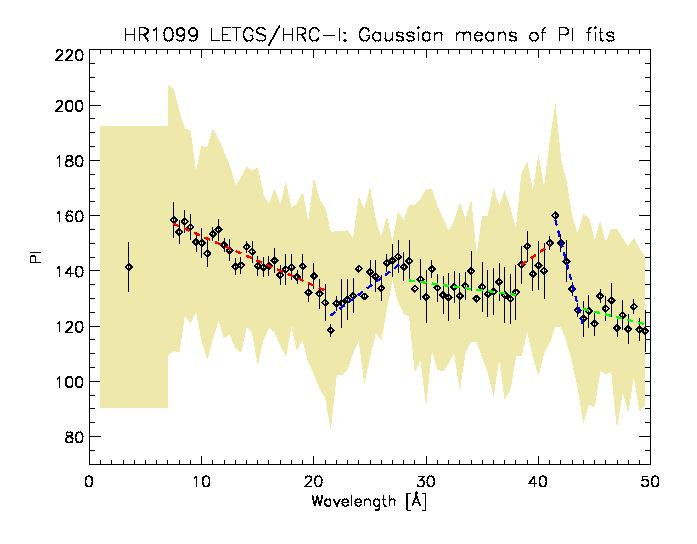Results of fitting wavelength "slices" of co-added HRC-I/LETG HR 1099 observations with Gaussians. The best-fits means are shown by diamond points, with one sigma error bars. The shaded region shows the best-fit Gaussian sigmas. The dashed lines show linear fits to the means. We constructed the RMF from these linear fits.

The HRC-I RMF can be used to calibrate hardness ratios or quantile color-color diagrams (QCCD) to distinguish between gross differences in the spectra. We recommend the QCCD, as it is relatively less dependent on temporal variations in the gain that are known to exist, and are not yet accounted for, in the gain-correction maps.

## Files

• Gain correction maps are needed to remove the spatial dependence of the gain variations across the HRC-I (Wilton et al. 2005) and to account for the gain drop since Oct 99 and generate PI values mimicking an on-axis observation. These maps are available in CALDB (see the HRC/Gain pages for details).
for observations carried out at the high-voltage setting, i.e., prior to 1999-oct-04:
\$CALDB/data/chandra/hrc/bcf/gain/hrciD1998-10-30gainN0002.fits
for observations carried out after the change to lower-voltage setting, i.e., after 1999-oct-04:
\$CALDB/data/chandra/hrc/bcf/gain/hrciD1999-10-04gainN0003.fits
\$CALDB/data/chandra/hrc/bcf/gain/hrciD2000-12-12gainN0002.fits
\$CALDB/data/chandra/hrc/bcf/gain/hrciD2002-01-26gainN0002.fits
\$CALDB/data/chandra/hrc/bcf/gain/hrciD2003-02-22gainN0002.fits
\$CALDB/data/chandra/hrc/bcf/gain/hrciD2004-11-25gainN0002.fits
\$CALDB/data/chandra/hrc/bcf/gain/hrciD2005-10-17gainN0002.fits
\$CALDB/data/chandra/hrc/bcf/gain/hrciD2006-09-06gainN0002.fits
Note: A series of AR Lac observations were carried out at multiple voltage settings, at various locations on the detector, on 1999-oct-04. To compute PI values for these observations, the appropriate gain file must be set manually during a run of hrc_process_events.

• Version 1 of the Response Matrix file (available in CALDB after December 2005):
\$CALDB/data/chandra/hrc/cpf/rmf/hrciD1999-07-22rmfN0001.fits
WARNING: Do not use for spectral fits; the spectral response is not sufficiently constraining to achieve a good fit with reasonable errors. If unavoidable, work in channel space (analysis channel), not energy space (analysis energy). Because the correspondence between PI bins and energy values is not well-defined and is not monotonic, the energy grid in the EBOUNDS extension of the RMF has been deliberately set to be meaningless (this matters only when spectra are plotted in energy space).

## Grids

Here we provide grids in hardness-ratio and quantile-width spaces for some common spectral models, as calculated using the HRC-I RMF derived above and a nominal on-axis ARF (v2.2). The grids were calculated using Sherpa. For the hardness ratio, we choose three bands in PI,

S == 1:100
M == 100:140
H == 140:255
and consider the colors
CSM=log10(S/M) and
CMH=log10(M/H).
As Park et al. (2006) point out, colors are the most well-behaved of the different types of hardness-ratios even at low counts.

For the quantile-width (see Hong et al. 2004) grids, we consider
the normalized median, m = Q50
and a measure of the width of the PI distribution, 3*(Q25/Q75),
where QX = (EX-E0)/(E100 - E0)
where EPP refers to the energy of the photon or the PI value of the event that corresponds to the PPth percentile in the observed spectral distribution (thus, E50 is the median). The quantile-width grids are more stable to temporal changes in gain than the hardness ratio grid.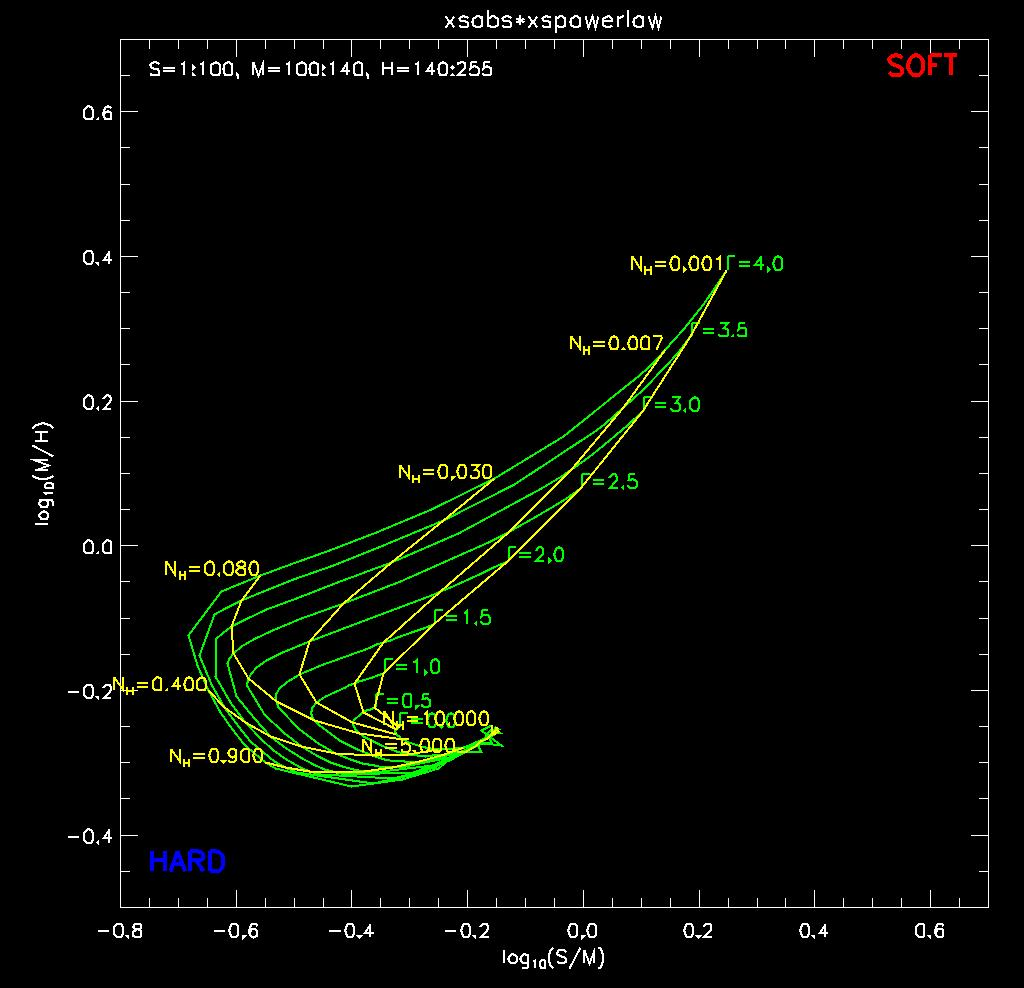The color-color grid for a powerlaw model, calculated for various values of the powerlaw index Gamma (green loci) and absorption column NH [1022 cm-2] (yellow loci).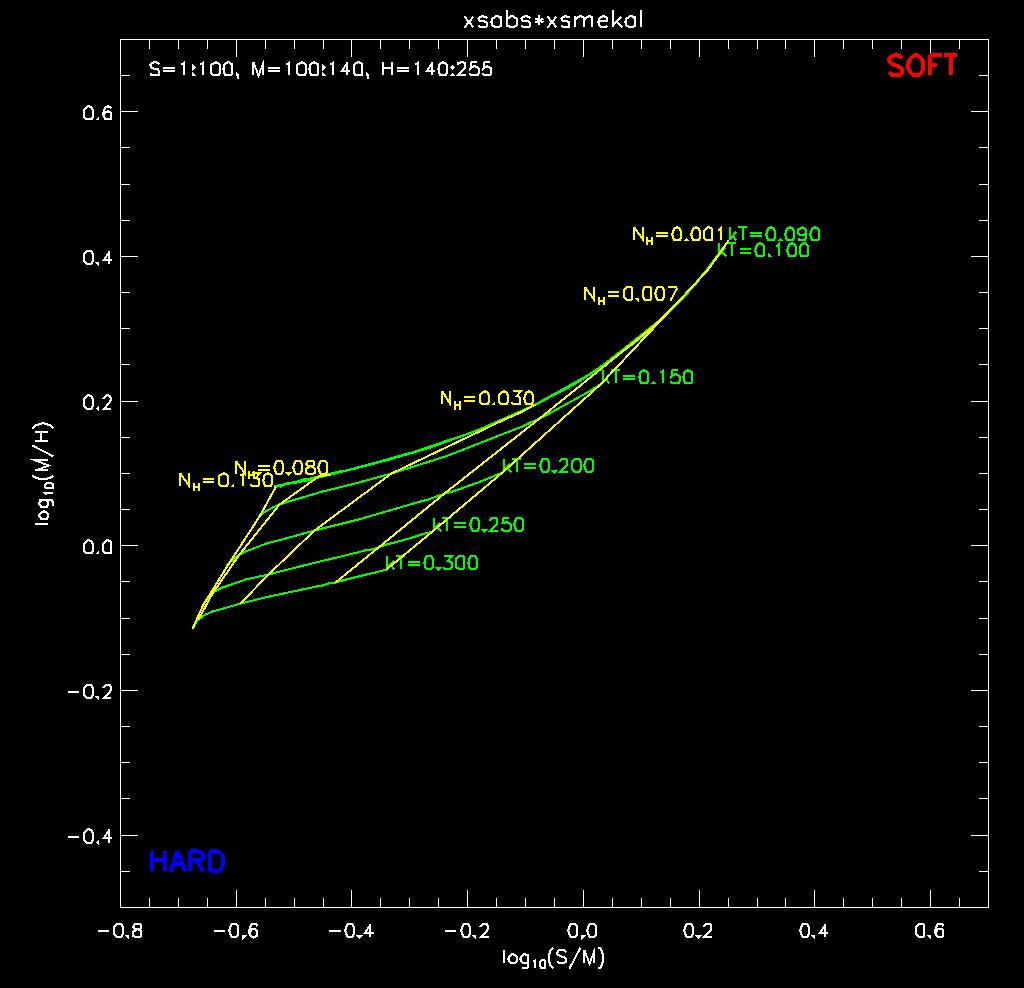The color-color grid for a MEKAL model, calculated for relatively low values of the plasma temperature kT [keV] (green loci) and absorption column NH [1022 cm-2] (yellow loci).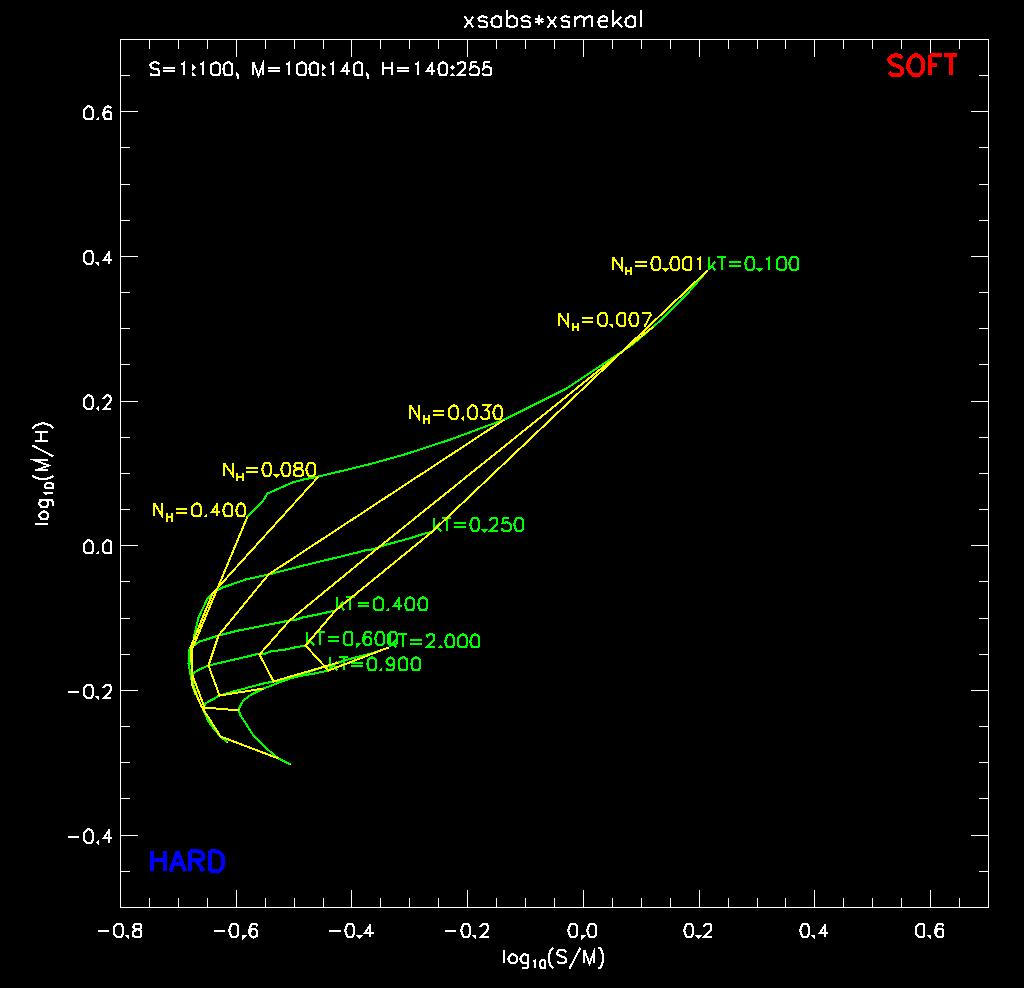The color-color grid for a MEKAL model, calculated for relatively high values of the plasma temperature kT [keV] (green loci) and absorption column NH [1022 cm-2] (yellow loci).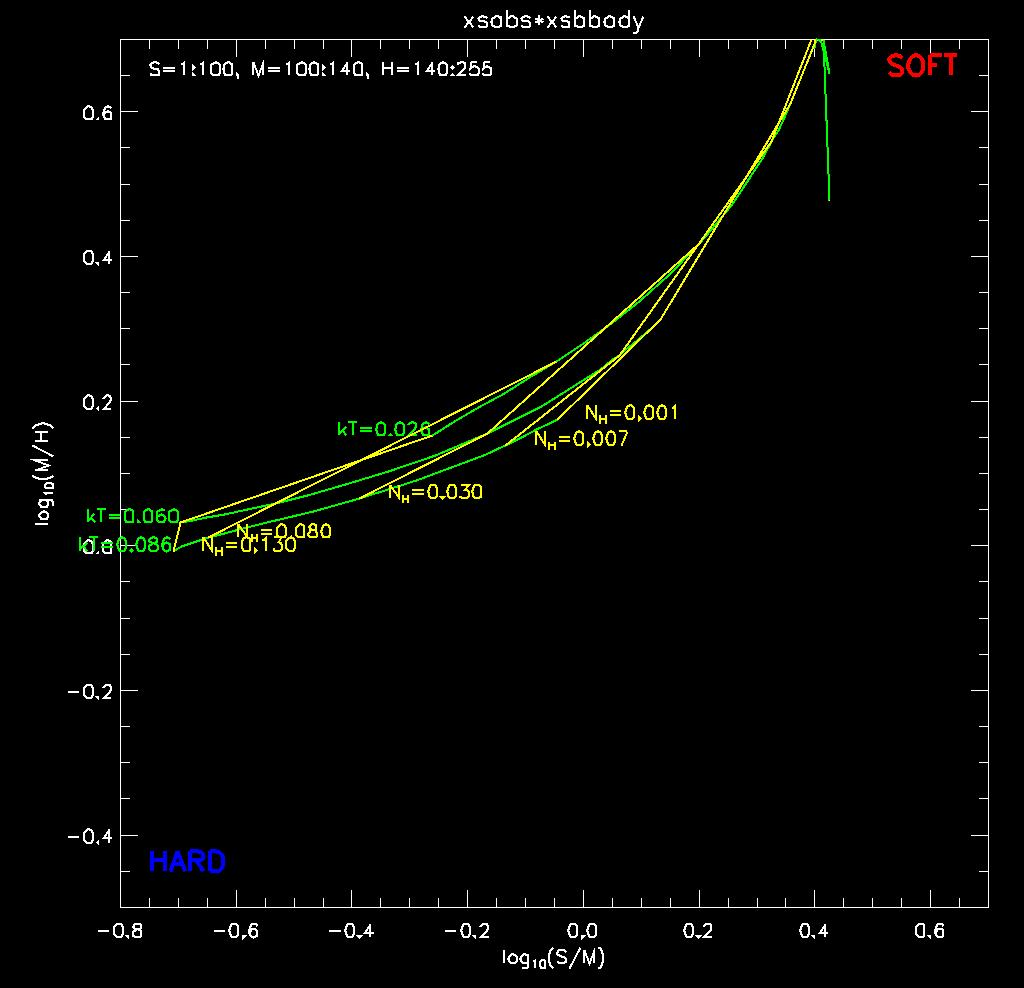The color-color grid for a Blackbody model, calculated for various values of the temperature kT [keV] (green loci) and absorption column NH [1022 cm-2] (yellow loci).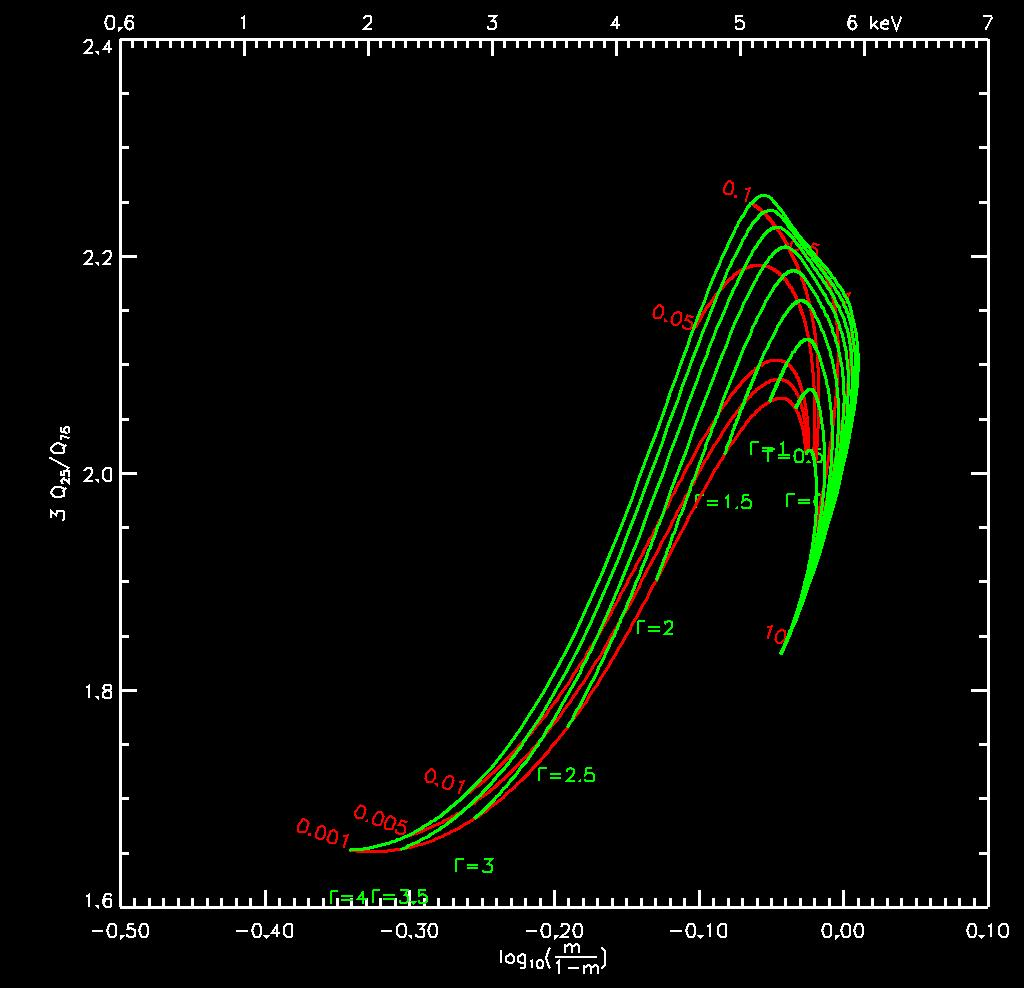The quantile-width grid for a powerlaw model, calculated for various values of the powerlaw index Gamma (green loci) and absorption column NH [1022 cm-2] (red loci).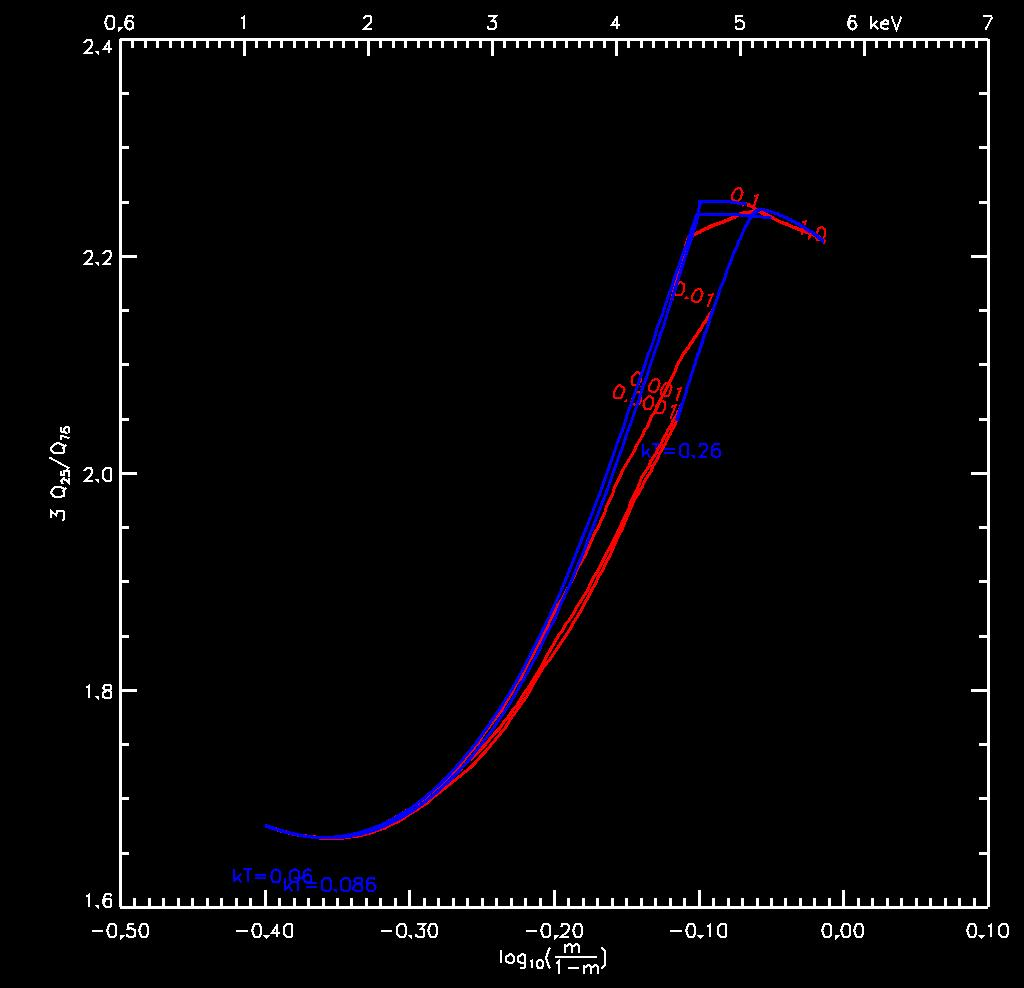The quantile-width grid for a MEKAL model, calculated for relatively low values of the plasma temperature kT [keV] (blue loci) and absorption column NH [1022 cm-2] (red loci).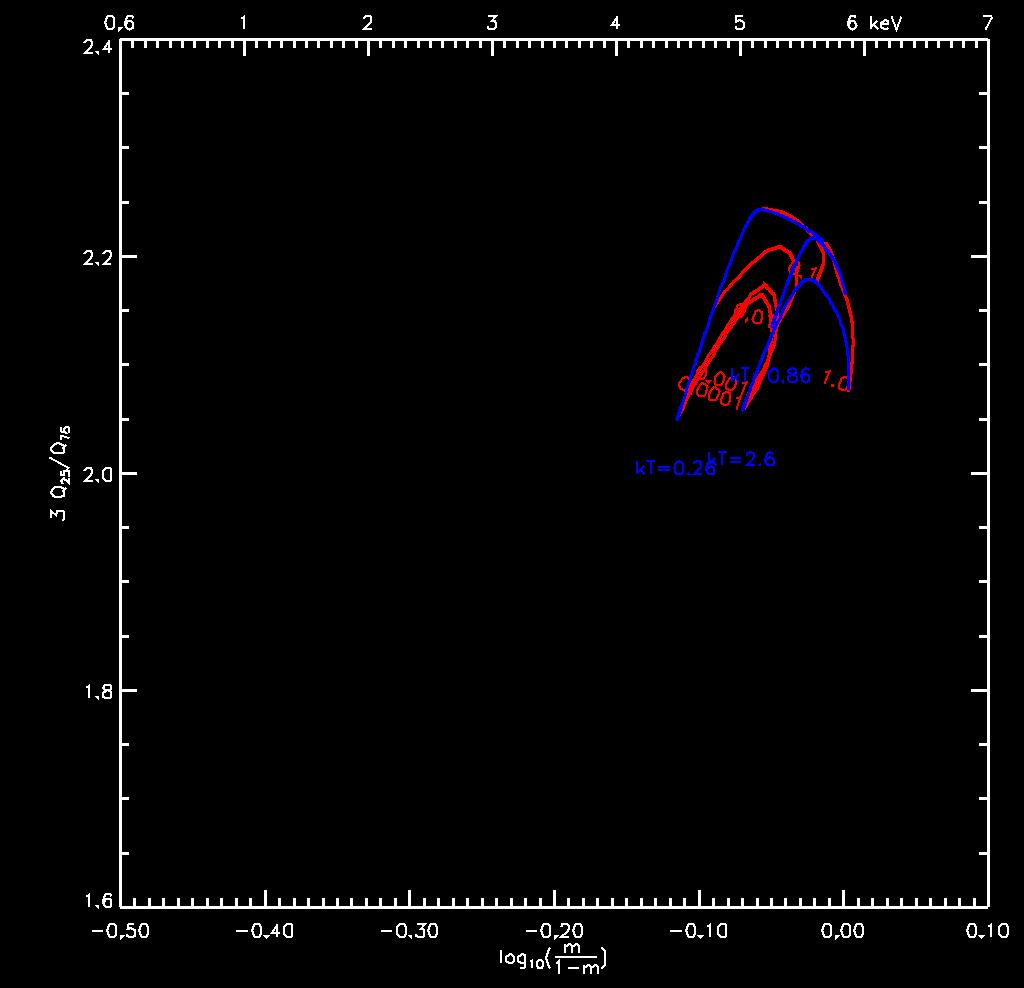The quantile-width grid for a MEKAL model, calculated for relatively high values of the plasma temperature kT [keV] (blue loci) and absorption column NH [1022 cm-2] (red loci).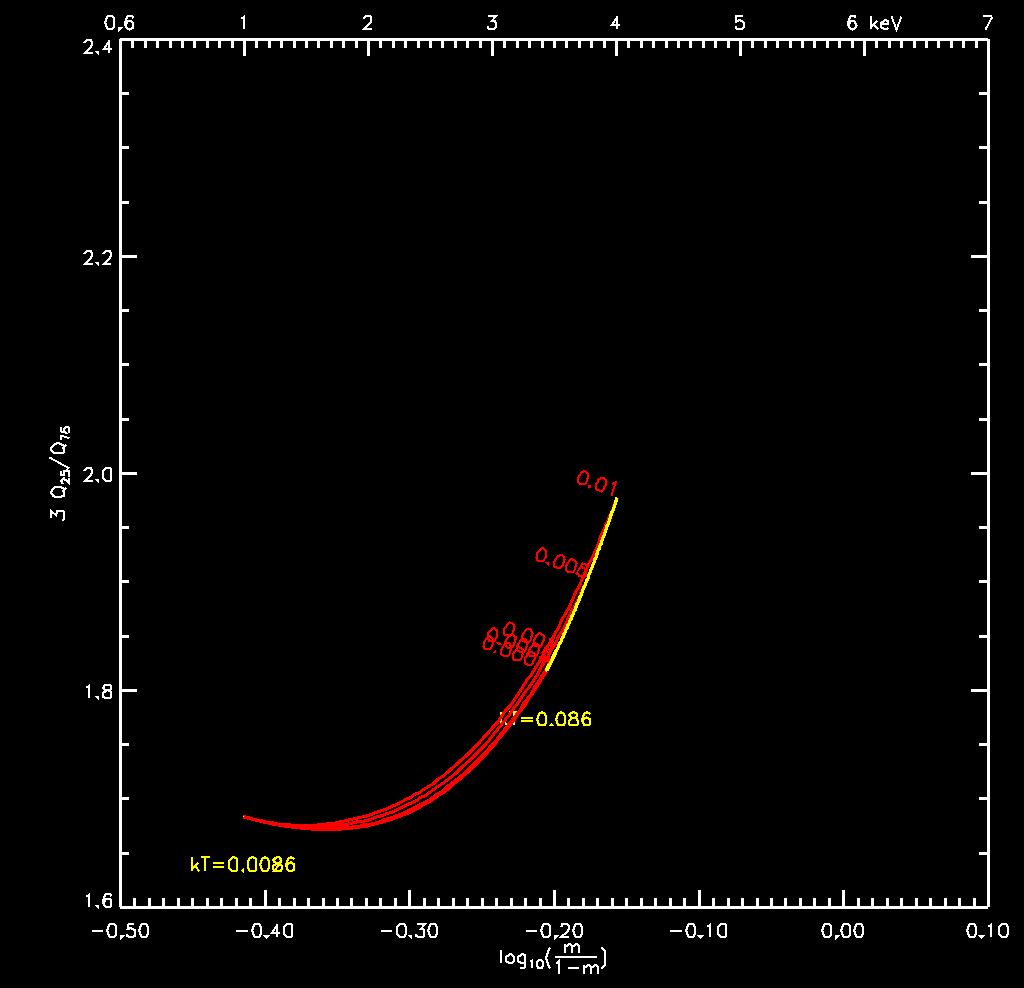The quantile-width grid for a Blackbody model, calculated for various values of the temperature kT [keV] (yellow loci) and absorption column NH [1022 cm-2] (red loci).

## HRC-S

#### Introduction | Files | Grids

Vinay Kashyap & Jennifer Posson-Brown
November 2006

## Introduction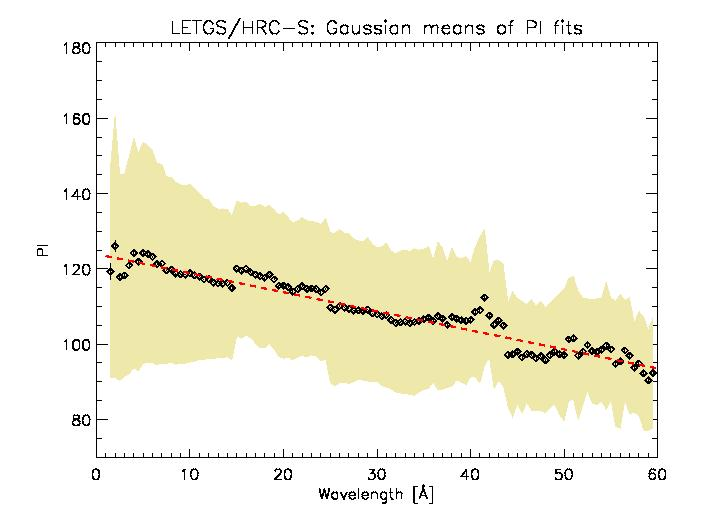Results of fitting Gaussians to wavelength "slices" of co-added HRC-S/LETG observations of RXJ1856, PKS 2155-304, Capella, V4743Sgr, and Mkn 421. The best-fits means are shown by diamond points, with one sigma error bars. The shaded region shows the best-fit Gaussian sigmas. The dashed line shows a linear fits to the means. We constructed the RMF from this linear fit.

## Files

• Gain correction maps are needed to remove the spatial dependence of the gain variations across the HRC-S and generate PI values mimicking an on-axis observation. These maps are available in CALDB (see the HRC/Gain pages for details).
\$CALDB/data/chandra/hrc/bcf/gain/hrcsD1999-07-22gainN0001.fits

• Version 1 of the Response Matrix file (available in CALDB after December 2006):
hrcsD1999-07-22rmfN0001.fits
WARNING: Do not use for spectral fits; the spectral response is not sufficiently constraining to achieve a good fit with reasonable errors. If unavoidable, work in channel space (analysis channel), not energy space (analysis energy). Because the correspondence between PI bins and energy values is not well-defined, the energy grid in the EBOUNDS extension of the RMF has been deliberately set to be meaningless (this matters only when spectra are plotted in energy space).

## Grids

Here we provide grids in hardness-ratio and quantile-width spaces for some common spectral models, as calculated using the HRC-S RMF derived above and a nominal on-axis ARF (v2.2). The grids were calculated using Sherpa. For the hardness ratio, we choose three bands in PI,

S == 1:80
M == 80:120
H == 120:255
and consider the colors
CSM=log10(S/M) and
CMH=log10(M/H).
As Park et al. (2006) point out, colors are the most well-behaved of the different types of hardness-ratios even at low counts.

For the quantile-width (see Hong et al. 2004) grids, we consider
the normalized median, m = (E50 - E0)/(E100 - E0)
and a measure of the width of the PI distribution, 3*(E25/E75),
where EPP refers to the energy of the photon or the PI value of the event that corresponds to the PPth percentile in the observed spectral distribution (thus, E50 is the median). The quantile-width grids are more stable to temporal changes in gain than the hardness ratio grid.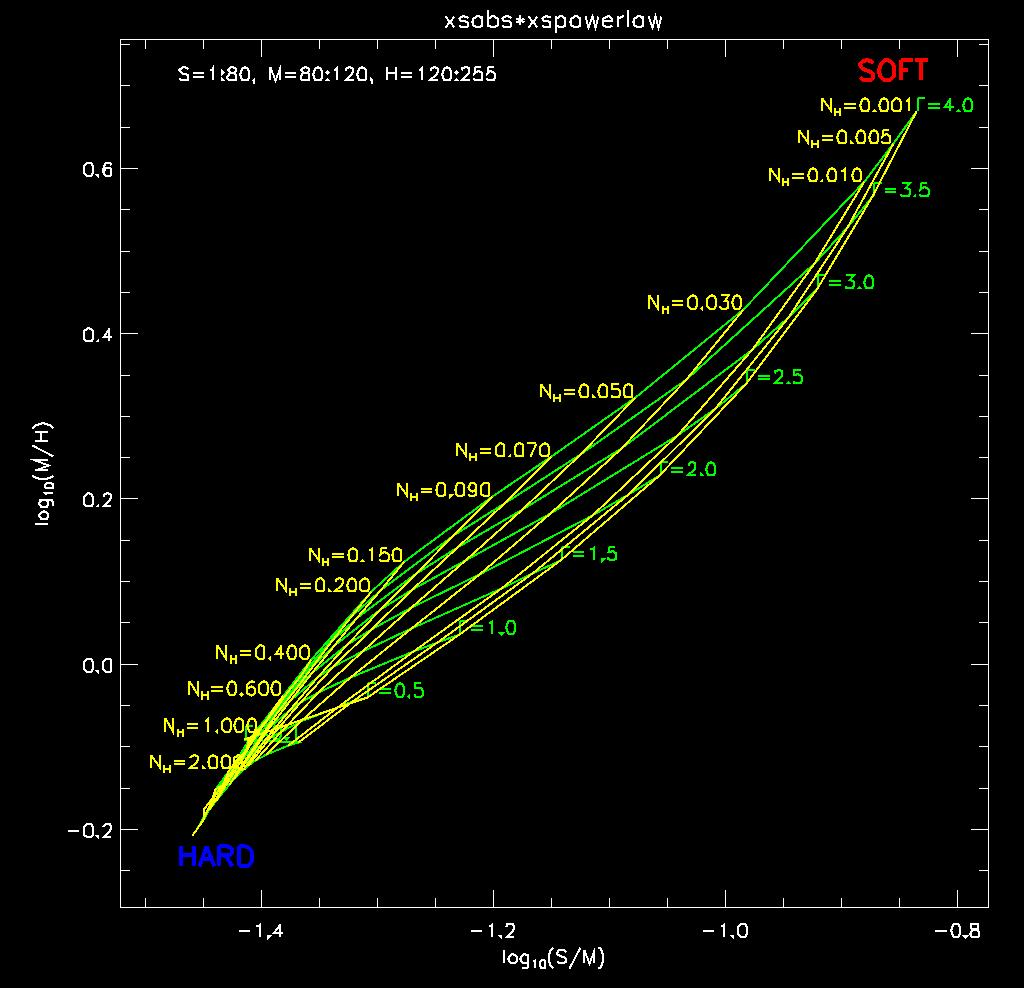The color-color grid for a powerlaw model, calculated for various values of the powerlaw index Gamma (green loci) and absorption column NH [1022 cm-2] (yellow loci).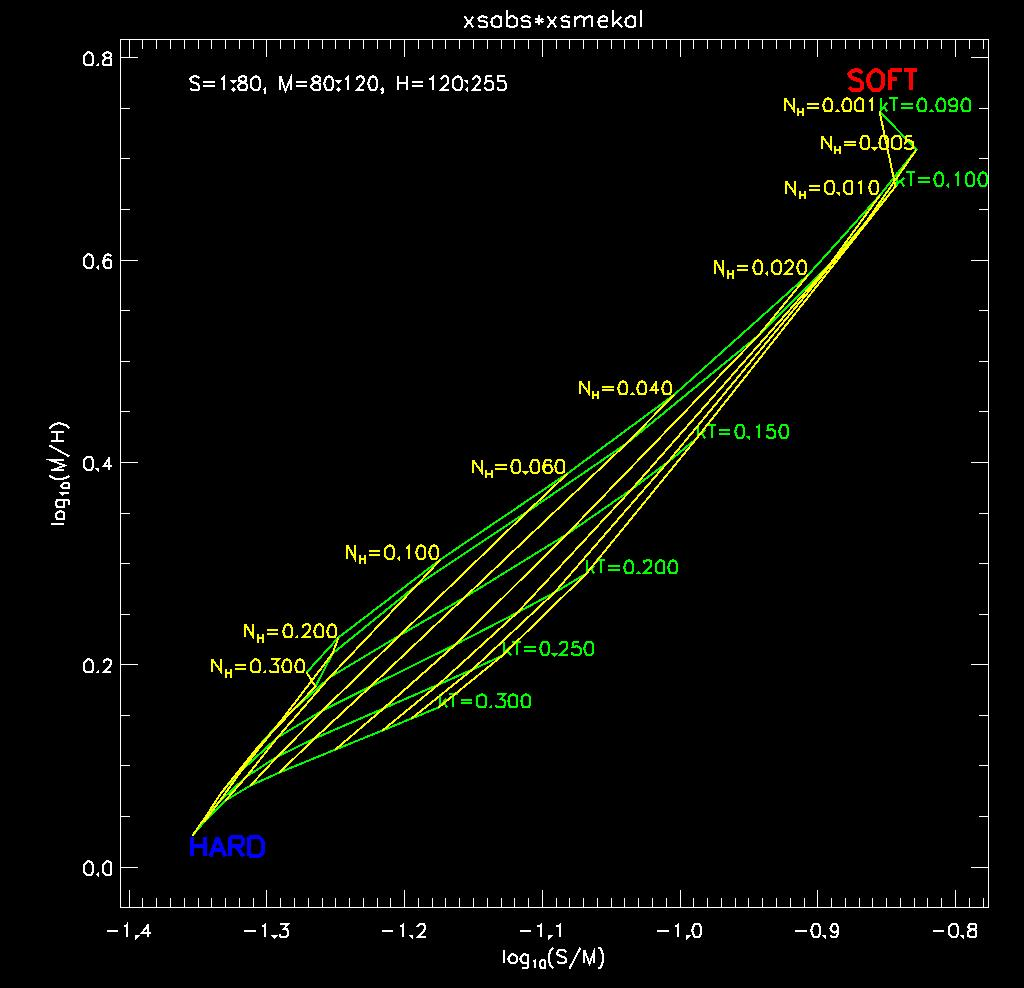The color-color grid for a MEKAL model, calculated for relatively low values of the plasma temperature kT [keV] (green loci) and absorption column NH [1022 cm-2] (yellow loci).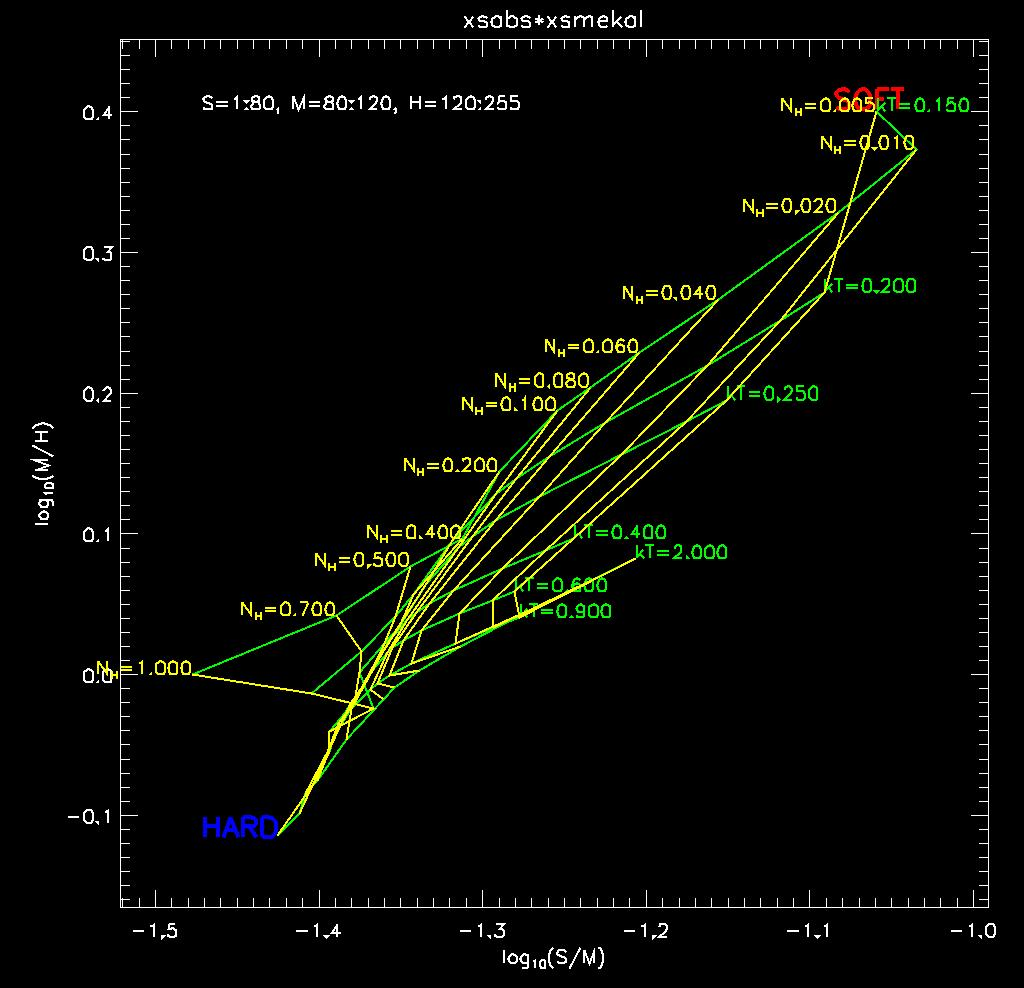The color-color grid for a MEKAL model, calculated for relatively high values of the plasma temperature kT [keV] (green loci) and absorption column NH [1022 cm-2] (yellow loci).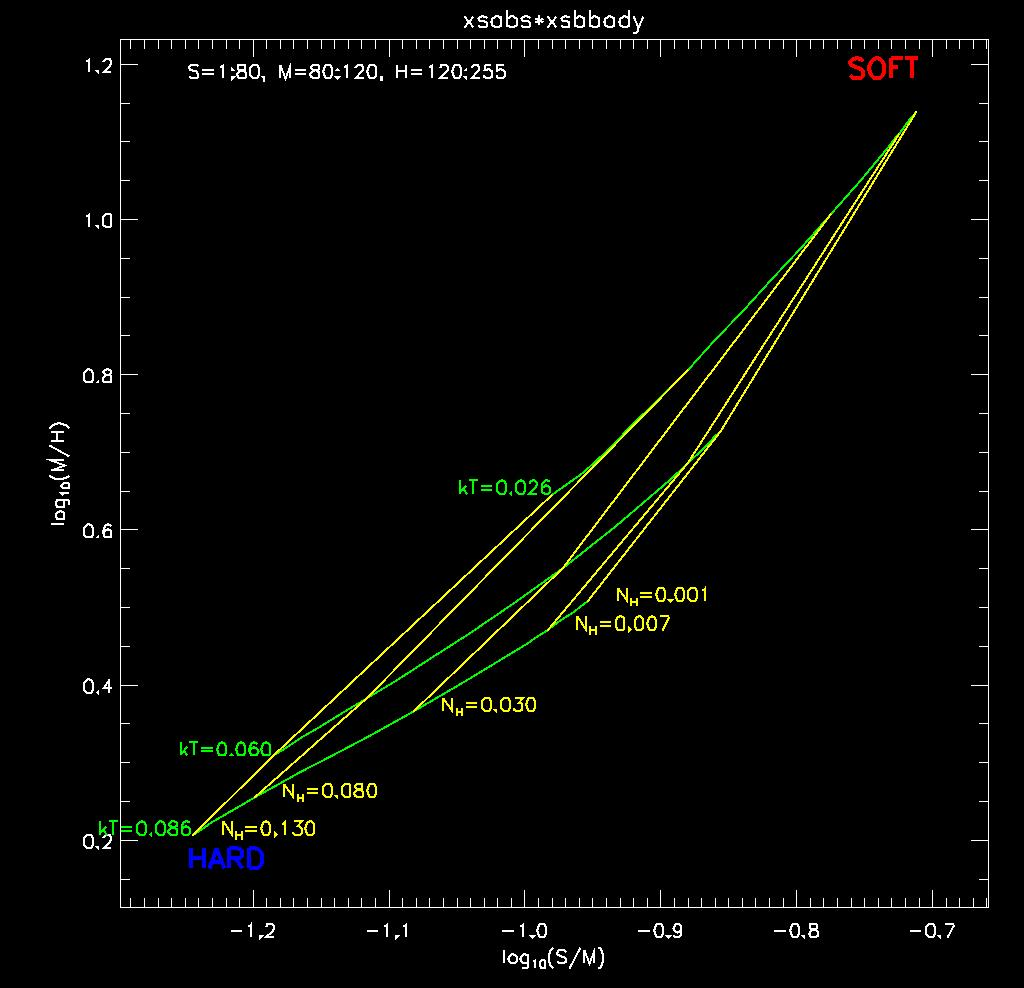The color-color grid for a Blackbody model, calculated for various values of the temperature kT [keV] (green loci) and absorption column NH [1022 cm-2] (yellow loci).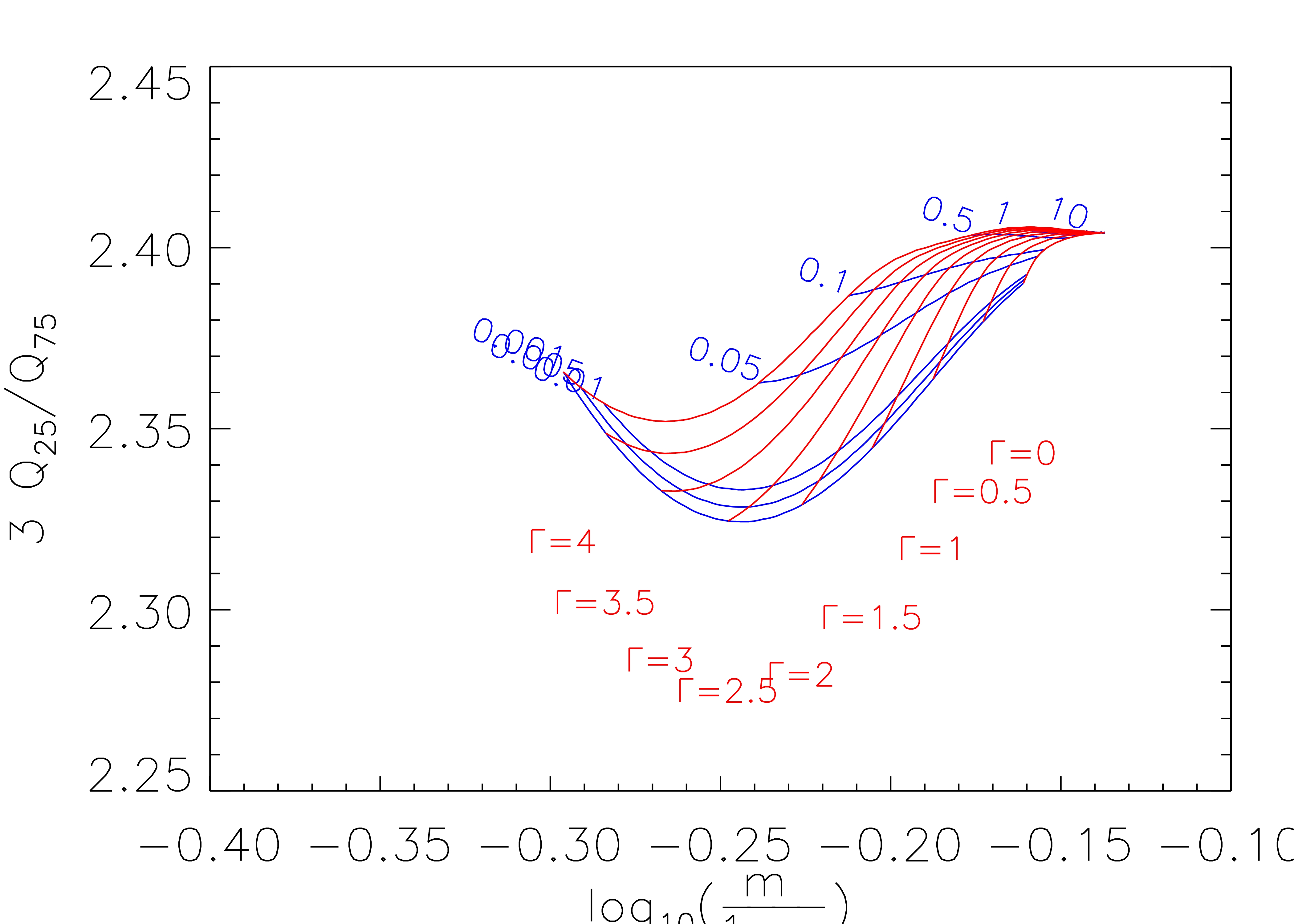The quantile-width grid for a powerlaw model, calculated for various values of the powerlaw index Gamma (red loci) and absorption column NH [1022 cm-2] (blue loci).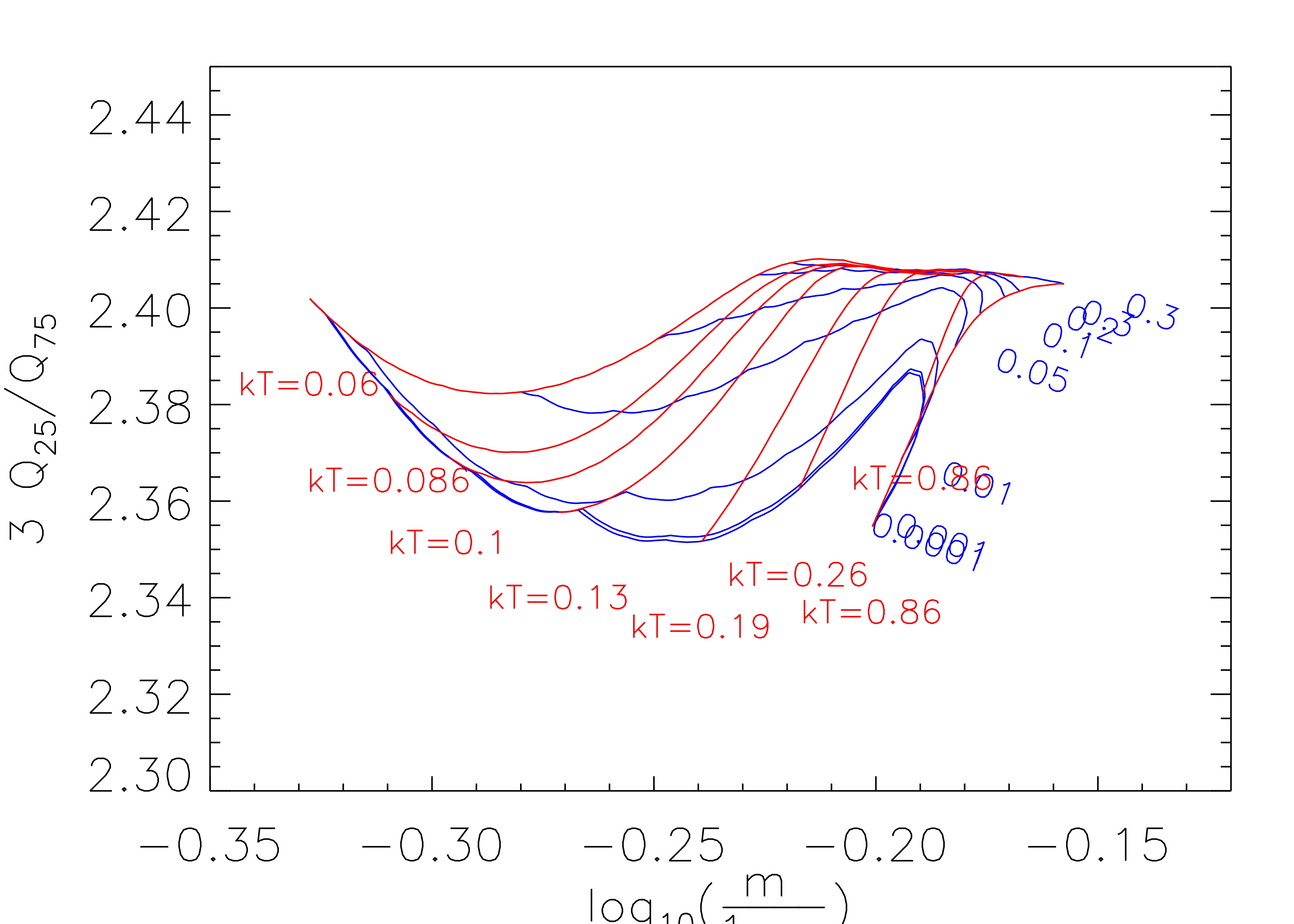The quantile-width grid for a MEKAL model, calculated for a variety of plasma temperature kT [keV] (red loci) and absorption column NH [1022 cm-2] (blue loci).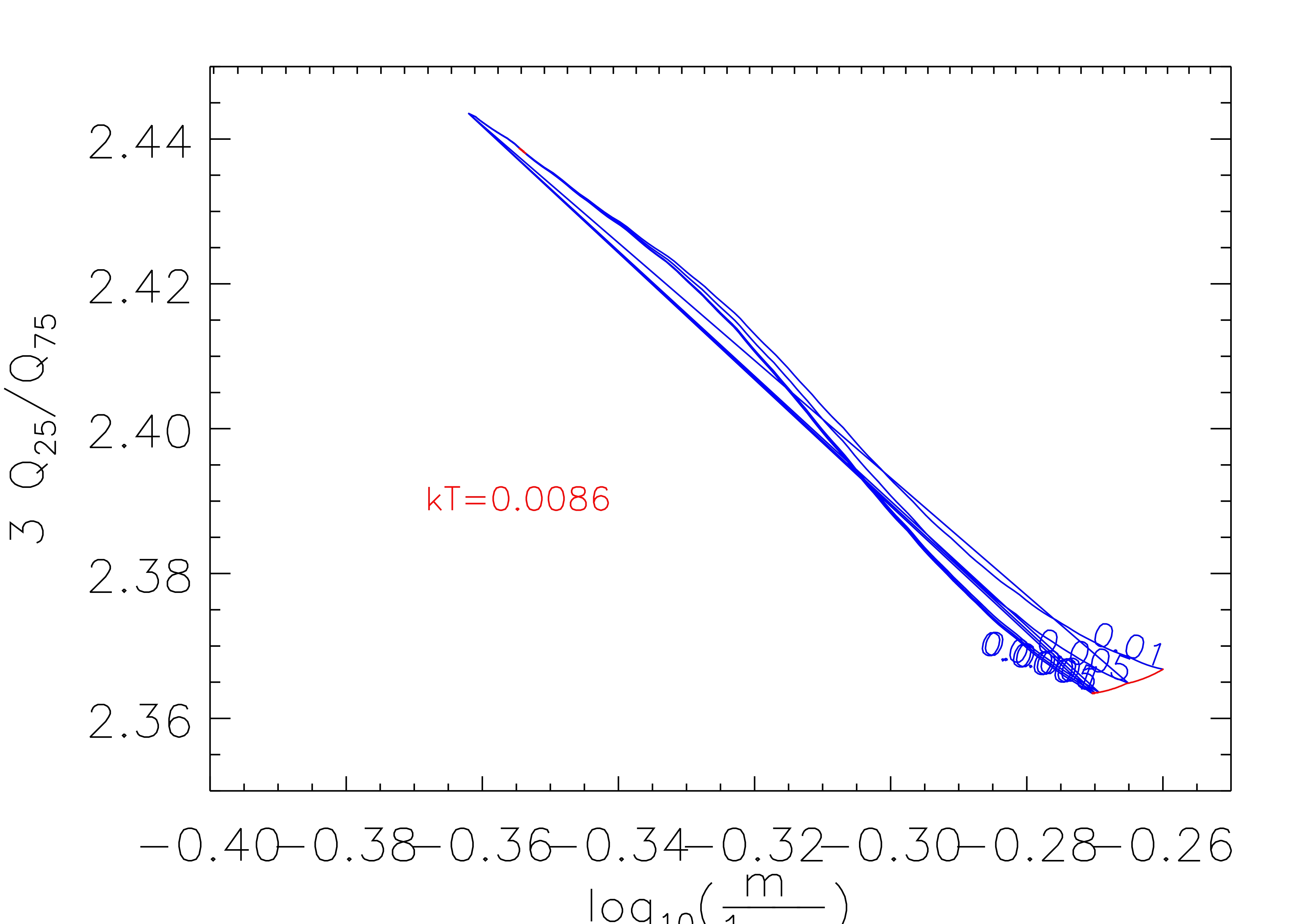The quantile-width grid for a Blackbody model, calculated for various values of the temperature kT [keV] (red loci) and absorption column NH [1022 cm-2] (blue loci).

We thank Hong Jae Sub, Doug Burke, and Andreas Zezas for assistance with generating the grids, Peter Freeman for useful discussions on the structure of RMFs, and Brad Wargelin for help with generating the HRC-S RMF.

## References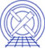The Chandra X-Ray Center (CXC) is operated for NASA by the Smithsonian Astrophysical Observatory. 60 Garden Street, Cambridge, MA 02138 USA.    Email: cxcweb@head.cfa.harvard.edu Smithsonian Institution, Copyright © 1998-2004. All rights reserved.